Physical Chemistry : Phase Changes

Example Questions

Example Question #21 : Thermochemistry And Thermodynamics

What amount of heat must be added to a 20-gram sample of ice in order to raise the temperature from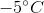to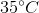?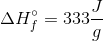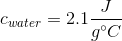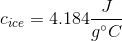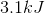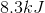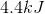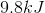Explanation:

This question requires three steps:

1. We need to raise the temperature of the ice fromtodegrees Celsius.

2. We need to melt the ice.

3. We need to raise the temperature of the water fromtodegrees Celsius.

Steps one and three can use the equation: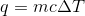Step 2 requires the enthalpy of fusion to be multiplied by the mass of the water sample.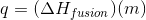Finding these three added heats will give us the total amount of heat needed to raise the temperature fromto.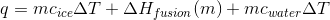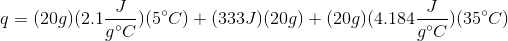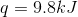All Physical Chemistry Resources